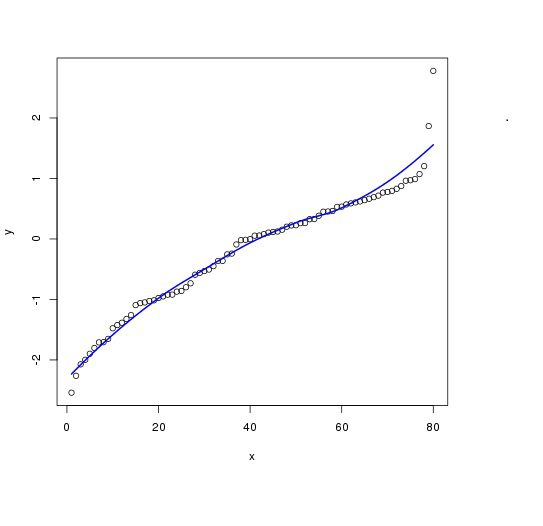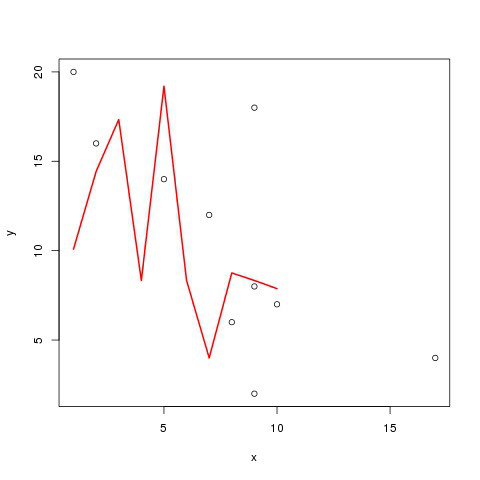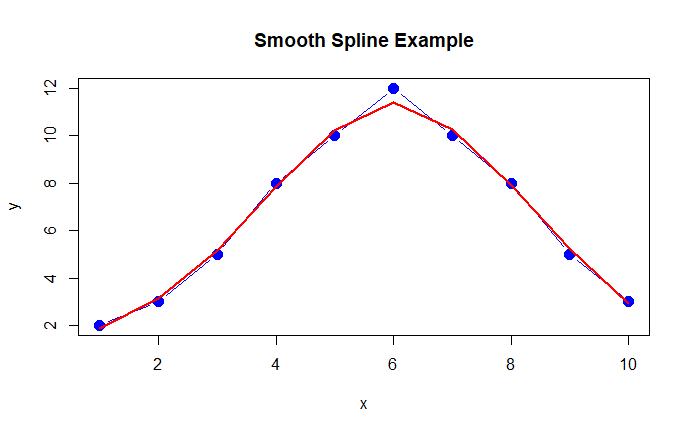GeeksforGeeks App
Open AppBrowser
Continue

# Fit Smooth Curve to Plot of Data in R

In this article, we will learn about the concept to fit a smooth curve to the plot of data in R Programming. Smoothing is an important concept in data analysis. It is also known as curve fitting and low pass filtering. The most common non-parametric method used for smoothing is loess() function. Loess is an abbreviation for Local Regression used to fit multiple regressions in the local neighborhoods. The span argument is used to control the size of the neighborhood. The size ranges between 0 and 1. The greater is the span value, the more smooth will be the fitted curve.

Use of Loess() function: Loess() function is used on a numerical vector to smoothen it. It is also used to predict the Y locally.

Syntax: loess(y ~ x)

Return: It smooth curve to plot of data

Example 1: Below is an implementation to fit a smooth curve to a plot:

## R

 `# Create example data``set.seed``(159632)            ``x <- 1:80``y <- ``sort``(``rnorm``(80))` `# Apply loess function``values <- ``loess``(y ~ x)   ``plot``(x, y)                     ``lines``(``predict``(values),``      ``col = ``"blue"``,``      ``lwd = 2)`

Output:In the above example loess() function is used to fit a smooth curve into plot. The lwd parameter is used to specify the line type of the smooth curve. The arguments x and y are used to provide coordination to the plot. The loess function will then set a smooth curve in the plot.

Example 2: Another example is illustrated using loess() function:

## R

 `x <- ``c``(7  ,5,  2,  9,  1,  9,  17,  8,  9, 10)``y <- ``c``(12, 14, 16, 18, 20, 2,  4,  6,  8,  7 )``values <- ``loess``(y ~ x)` `plot``(x, y)``lines``(``predict``(values), col = ``'red'``, lwd = 2)`

Output:In the above example, X and Y axis values are plotted. The smooth curve is fitted using the loess function. The prediction will be an array of the appropriate dimensions in a smooth fitted curve.

Example : The fit a smooth curve to a plot of data using the smooth.spline() function.

## R

 `# Generate some data``x <- 1:10``y <- ``c``(2, 3, 5, 8, 10, 12, 10, 8, 5, 3)` `# Create a plot of the data``plot``(x, y, type = ``"b"``, col = ``"blue"``, pch = 16, cex = 1.5,``     ``main = ``"Smooth Spline Example"``, xlab = ``"x"``, ylab = ``"y"``)` `# Fit a smooth spline to the data``fit <- ``smooth.spline``(x, y)` `# Add the smooth curve to the plot``lines``(fit, col = ``"red"``, lwd = 2)`

Output :The generate some data for the plot by creating two vectors, x and y. x contains the values 1 through 10, and y contains some arbitrary values for demonstration purposes.

My Personal Notes arrow_drop_up